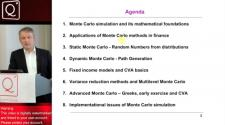# Monte Carlo Simulation in Finance (Part 1)

00:00Monte Carlo Simulation in Finance: Models, Algorithms and Practice with Application to Derivatives Pricing, Risk Measures and CVA (Part 1)

Presenter: Jörg Kienitz: Head of Quantitative Analysis, Treasury, Deutsche Postbank

Course Running Time: 6 Hours 30 Minutes

Session 1: (Running Time: 08:08)

Session 2 (Running Time: 1:15:00)

Session 2a: (Running Time: 1:08:00)

Session 3 (Running Time: 1:36:16)

Session 4: (Running Time: 1:31:57)

Session 5 (Running Time: 1:08:52)

Summary:

The goal of this two day seminar is to provide a detailed overview, offering insights into the latest techniques of modeling uncertainty in financial markets and demonstrating computational methods to tackle the industry applied models. We show the applicability of Monte Carlo simulation to derivatives pricing, risk measurements or CVA calculation.

We explain how the basic method is set up and we discuss the main ingredients. Finally, we discuss methods for improving and speeding up the method as well as recent techniques for calculating Greeks.

Each single topic is illustrated using Matlab code (No Laptops are needed for this event, however delegates will be given example sheets) .

The seminar is an ideal way to get a detailed overview of Monte Carlo methods and how to apply such techniques to problems arising in finance.

Main Topics:

This seminar discusses the application of Monte Carlo simulation to financial problems. Problems include scenario generation, risk measures, derivatives pricing or CVA calculation. The bullet points are:

• Probability Theory and Stochastic Processes
• One and multifactor models
• General purpose and special sampling schemes for e.g. Heston or SABR models
• Static and dynamic Monte Carlo Methods
• Risk Measures via scenario generation
• Derivatives pricing and hedging strategies
• Interest rate simulation and CVA
• Calculating sensitivities (‘Greeks’) and early exercise rights
• Implementing Monte Carlo methods

Methods:

• Presentation (slides)
• Illustration using computer examples

Prerequisites:

To participate in this course, you need to have a basic background in stochastic modelling but all the concepts and models are introduced and discussed in detail.

Day 1 Topics:

Mathematical Basics

• Foundations of Probability
• How does Monte Carlo Work?
• Distributions
• Basic Distributions in Finance
• Stochastic Processes
• Diffusion Processes
• Jump-Diffusion Processes
• Jump Processes

Applications of the Monte Carlo Method

• Option Pricing
• Evaluating Hedge Strategies
• Scenario Generation and Risk Measures

Static Monte Carlo Simulation

• Sampling from the Uniform Distribution
• Random Number Generators
• Good ones and bad ones
• Sampling Techniques
• Inverse Method
• Ratio of Uniforms
• Sampling from the Normal and other Distributions

Dynamic Monte Carlo Simulation

• Path Generation Methods
• (Log) Euler-Scheme
• Predictor Corrector
• Bridge Sampling
• Exact Sampling
• Sampling from Jump Diffusion Processes
• SGS Sampling
• FGS Sampling
• Sampling from Pure Jump Processes
• Variance Gamma, NIG
• Stochastic Volatility Lévy Models

Implementation Issues (from Algorithms to Code I)

• Ingredients for a successful implementation of Monte Carlo algorithms
• Object Oriented Design I

Monte Carlo

7 March 2014

£99.00

## Presenter BioJörg Kienitz

Jörg Kienitz: Director, Financial Risk Solutions, FSI Assurance, Deloitte & Touche GmbH. Previously: Head of Quantitative Analytics, Dt. Postbank AG, Senior System Architect, Postbank Systems AG Financial Consultant, Reuters Academic: PhD Math, Diploma Math Books (Wiley): (A) Monte Carlo Frameworks in C++ (B) Financial Modelling - Theory, Implementation and Practice with Matlab Code

## Related workshops### Alexander Sokol

#### XVA: Long Term Portfolio Simulation for CVA, Funding, Limits, and Capital

7 March 2014### Jörg Kienitz

#### Monte Carlo Simulation in Finance (Part 2)

7 March 2014### Roger Lord

#### Interest Rate Modelling: Pricing Options via Fourier Inversion & Simulation of Stochastic Volatility Models

14 March 201423 May 2016